Rectilinear motion is termed as the motion of particles of a rigid body such that each of them travels in a straight line and parallel to each other. Moreover, they travel the same distance as compare to one-another in the same direction.

Suppose the mass of one particle is m travels in x direction. The force applied in the particle is F in the same direction then,

According to Newton’s 2nd law of motion, distance travelled by the particle due to the action of external force is given by,

x = m. ax                             (This is a scalar product in x-direction)

Where,

ax = acceleration in x-direction

Considering the force in y-direction and z-direction,

y = 0

z = 0Let us consider the particle has three components of acceleration such that ax, ay and az. In this case, force can be written as,

x = m. ax

y = m. ay

z = m. az

To calculate the value of acceleration, we determine,

= ax+ ay+ az

||= a = (ax2 + ay2 + az2)

Similarly,

Force, = x +y+ z

||=F = (Fx2 + Fy2 + Fz2)

The S.I. Unit of Force is

1 Newton (N)   = 1 kg x 1 m/ s2

= 1 kgm/ s2

Example 5.1:

Suppose a body was in rest when an external force is acted on it. The force on the body produces 6 m/s2 of acceleration to give it some motion. If the mass of the object is 15 kg, then calculate the magnitude of force.

Solution:

Given Data:

Acceleration, a = 6 m/s2

Mass, m = 15 kg

We know that,

Force, F = m . a

= 15 . 6

= 90 N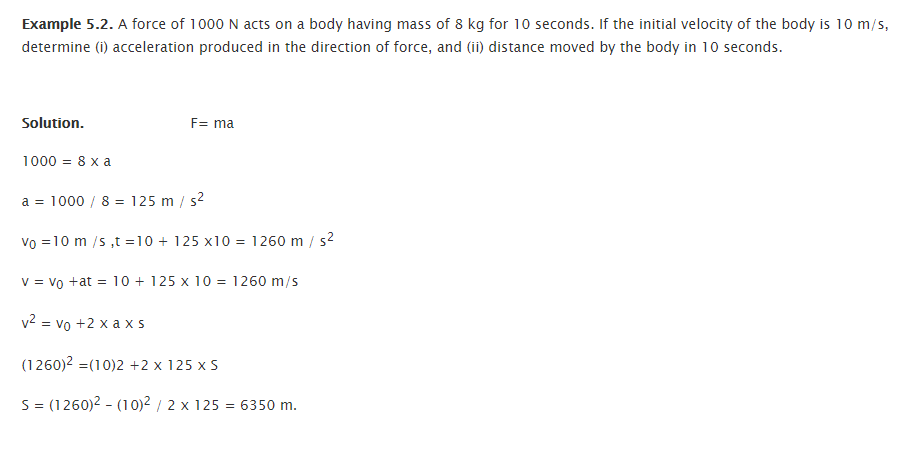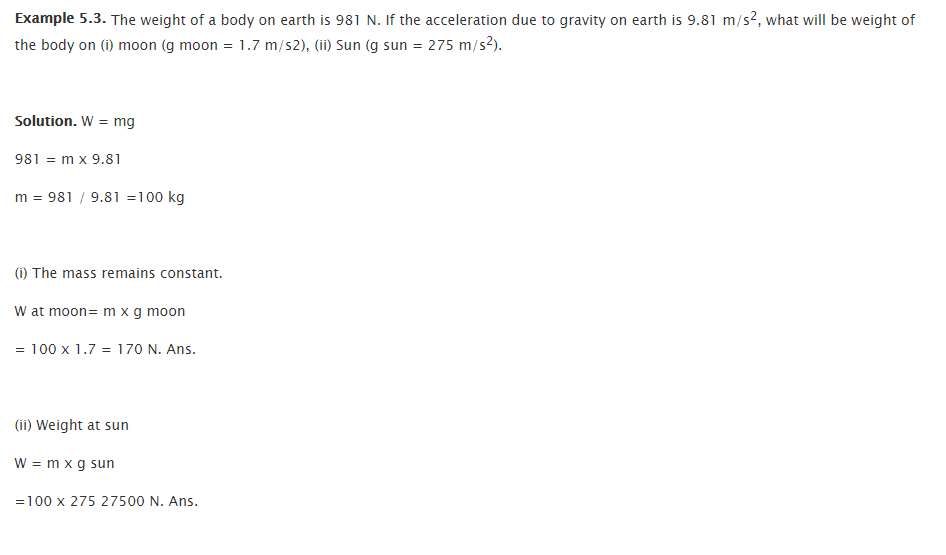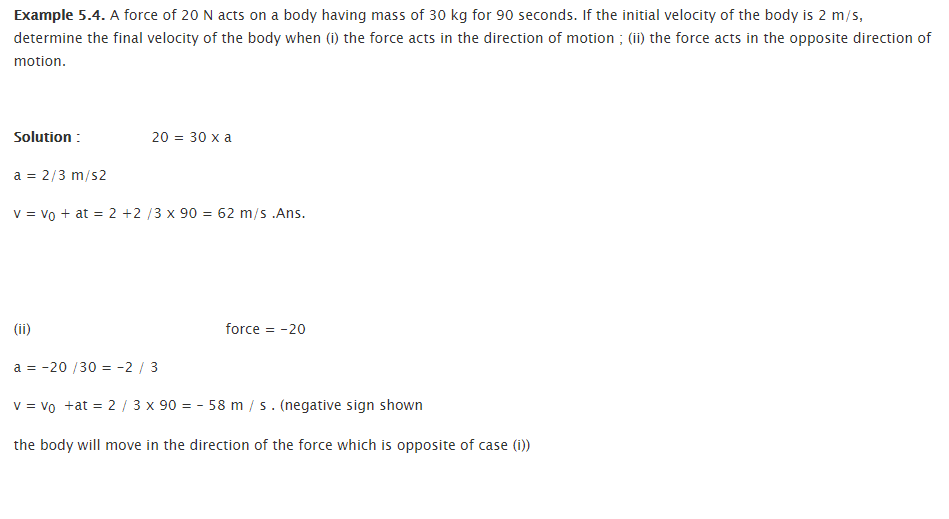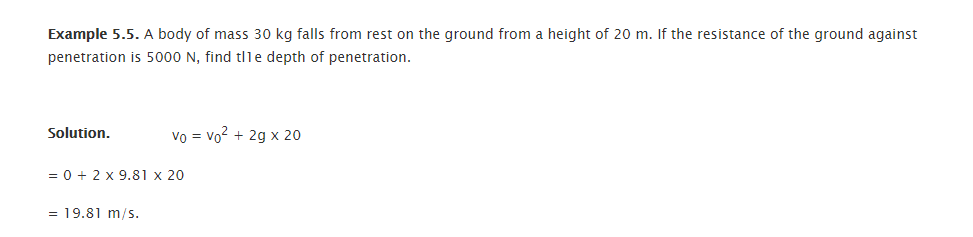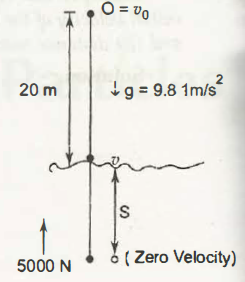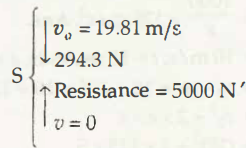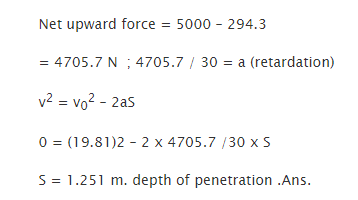Links of Next Mechanical Engineering Topics:-### Customer Reviews

My Homework Help
Rated 5.0 out of 5 based on 510 customer reviews at